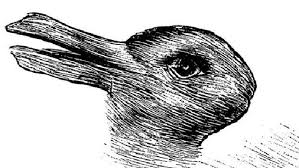## Middle School Math Post – Week of April 22-26, 2019Happy Earth Day!

6th Grade – Section A – Mrs. VonFeldt

This week we are continuing to write equations in two variables. We will look at how to graph these relationships on a coordinate plane and find solutions on the graph. This is a preview into the algebraic study of linear equations. There will be a Chapter 9 test on Friday.

6th Grade – Section B – Mrs. Evans

This week our section of 6th grade will continue working on Chapter 9 in which we will be extending our understanding of writing equations to writing equations with a dependent and independent variable, translating between equations and tables and graphs.  We will do problem solving using these tools to make conclusions.

IXL has excellent practice –TWO VARIABLE EQUATIONS – BB.1 through BB.10

Pre Algebra (7th and 8th Grade) – Mrs. Evans

Pre-algebra continue in our studies of statistics and probability!  This week, we are comparing data from multiple dot and box plots.  We are learning to make inferences (predictions) about the populations from the plots and also by using proportional reasoning.

For fun, here is another optical illusion reminding us that we have to be very cautious in determining whether we are biased or not.  What do you see in these pictures?8th Grade Math – Mr. Pryor

Eighth grade math is almost halfway through chapter 8. We are graphing now in standard and slope-intercept form and looking at manipulations.

Algebra – Mrs. VonFeldt

Students continue to solve quadratic equations. They now have four strategies to use: Factoring, Graphing, Completing the Square, and the Quadratic Formula. At the end of the week we will expand our study to include solving systems of quadratic equations. The test will be next week.

## Middle School Math – Holy Week

Blessings on your Holy Week!

6th Grade – Section A – Mrs. VonFeldt

This week we will begin Chapter 9. In this chapter, we analyze relationships between two variables in an equation, where one variable is dependent on the other. Students will begin by creating input/output tables and looking for patterns in the data.

6th Grade – Section B – Mrs. Evans

This week the 6th grade will be spending most of the week at Camp Hamilton but they will also continue learning about algebra as they create equations with two variables – one dependent on the other.  These lessons are in Chapter 9.

For those having some difficulty with the distributive property – please visit this link:

Using the Distributive Property

Pre Algebra (7th and 8th Grade) – Mrs. Evans

Prealgebra has begun to study statistics and probability!  We are investigating how to create random samples of populations and how represent the data when shown in dot and box plots.  We are learning to make inferences (predictions) about the populations from the plots and also by using proportional reasoning.                                                                                                                                                           We are becoming aware of the bias we have that inhibits us from being able to make random selections.  For a fun example – look at the circles below – come to class with your guess – which of the orange circles are bigger or are they are the same size?8th Grade Math – Mr. Pryor

Eighth grade math is well into chapter 8. We are studying how to graph slope-intercept equations, starting with finding slope (m).

Algebra – Mrs. VonFeldt

Which function is a pineapple pizza and which is a pizza pinapple?In Chapter 9 we are focusing on solving quadratic functions. This week we will look at how to determine a solution using a graph, using square roots, and finally using the quadratic formula. To review factoring, use the Khan Academy link below.

Khan Academy: Factoring by Grouping